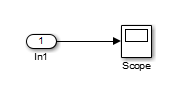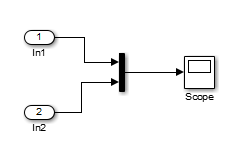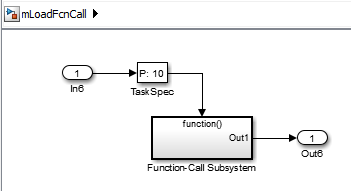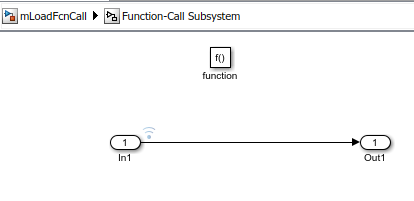## Load Data to Root-Level Input Ports

You can load data from a workspace to a root-level inport modeled using one of these blocks:

• Inport block

• Enable block

• Trigger block that has an edge-based (rising, falling, or either) trigger type

These blocks import data from the workspace based on the value of the Configuration Parameters > Data Import/Export > Input parameter.

Tip

To import many signals to root-level input ports, consider using the Root Inport Mapper tool. For more information, see Map Root Inport Signal Data.

You can also import data from a workspace using a From Workspace block. For details, see the From Workspace documentation and Load Data for an Input Test Case.

### Specify Input Data

You can specify input data manually, using the Input configuration parameter. To load many signals to root-level input ports, consider using the Root Inport Mapping tool, which automatically specifies in the Input parameter the data you map using the tool. For details, see Map Data Using Root Inport Mapper Tool.

1. Select the Configuration Parameters > Data Import/Export > Input parameter.

Note

The use of the Input configuration parameter is independent of the setting for the Format configuration parameter for saving logged data.

2. Enter an external input specification in the adjacent edit box and click . For a list of the forms of data you can specify, see Forms of Input Data.

In the Input box, specify the signal input using one of these approaches:

• Create data at run-time for each simulation time step using the input ```u = UT(t)``` for either a MATLAB® function (expressed as a string) or MATLAB expression.

• Specify the data directly, using one of the input data forms described in Forms of Input Data.

#### Comma-Separated List

If you specify `Dataset` data, specify only one `Dataset` object for the Input parameter. Do not include it in a comma-separated list.

Each variable or expression must evaluate to an appropriate object that corresponds to a specific root-level input port in the model. Each variable or expression in the list must evaluate to the appropriate object that corresponds to one of the root-level input ports of the model. The first item corresponds to the first root-level input port, the second to the second root-level input port, and so on. The dimensions for each data sample must match the dimensions of the data specified in the input block parameter.

For an Enable or Trigger block, the signal driving the enable or trigger port must be the last item in the comma-separated list. If you have both an enable and a trigger port, then specify:

• The enable port as the next-to-last item in the list

• The trigger port as the last item

Use an empty matrix to specify ground values for a port. For example, to load data for input ports `in1` and `in3`, and to use ground values for port `in2`, enter the following in the Input parameter:

`in1, [], in3`

### Forms of Input Data

You can provide input data with the following formats:

Note

When you specify `timetable` data to load, the `timetable` must contain data for only one signal.

For information about importing bus data, see Load Bus Data to Root-Level Input Ports.

### Time Values for the Input Parameter

Time values that you specify in the Input parameter do not control the times the solver uses. Solvers have their own logic for propagating time and might require input data at an arbitrary time value. The Interpolate parameter setting for the root-level input block (for example, the root-level Inport block) specifies how to handle output at time steps for which no corresponding workspace data exists.

The time values specified in the Input parameter cannot be sparse or include `NAN` or `Inf` values.

If you select the Interpolate data option for the corresponding Inport, Enable, or Trigger block, Simulink® linearly interpolates or extrapolates input values as necessary.

Simulink resolves symbols used in the external input specification as described in Symbol Resolution. The `sim` command provides some data import capabilities that are available only for programmatic simulation.

If you use a `Simulink.SimulationData.Dataset` object that includes a `matlab.io.datastore.SimulationDatastore` object as an element, then the data stored in persistent storage is streamed in from a file. For more information, see Load Big Data for Simulations.

You can use a Dataset object as a value for the Configuration Parameters > Data Import/Export > Input parameter. Specify only one `Dataset` object and do not include it in a comma-separated list. The number of elements in the `Dataset` must match the number of root-level input ports.

#### Dataset Elements

A `Dataset` object can include elements with different data types.

For individual non-bus signal data, you can specify these types of data for `Dataset` elements:

• `timeseries`

• `timetable`

• `matlab.io.datastore.SimulationDatastore`

• `double` vectors or structure of `double` data

• a `Simulink.SimulationData.Signal`, `Simulink.SimulationData.State`, or `Simulink.SimulationData.DataStoreMemory` object

• An array that meets one of these requirements:

• An array with time in the first column and the remaining columns each corresponding to an input port. See Loading Data Arrays to Root-Level Inputs.

• An `nx1` array for a root inport that drives a function-call subsystem.

For bus signals, use a structure with a data element for each leaf signal, using one of these formats:

• A MATLAB `timeseries` object

• A MATLAB `timetable` object

• A `matlab.io.datastore.SimulationDatastore` object

• An empty matrix

• Another structure, with data elements for each signal that are consistent with these requirements for a structure for bus data

Note

When you specify `timetable` data to load, the `timetable` can contain data for only one signal.

#### Create a Dataset Object for Inport Blocks

To generate a `Simulink.SimulationData.Dataset` object from the root-level Inport blocks in a model, you can use the `createInputDataset` function. Signals in the generated dataset have the properties of the Inport blocks and the corresponding ground values at model start and stop times. You can create `timeseries` and `timetable`objects for the time and values for signals for loading. The other signals use ground values. Each `timetable` object must contain data for only one signal.

You can load into a root-level input port data specified by a MATLAB `timeseries` object that resides in a workspace.

Note

This documentation about importing MATLAB timeseries data includes examples of root Inport blocks. Unless specifically noted otherwise, the examples are applicable to root-level Enable, Trigger, and From Workspace blocks.

#### Time Dimension

When you create a MATLAB `timeseries` object to import data to Simulink, the time dimension (number of time samples) depends on the dimension and the type of signal data.

Signal Data Dimension or TypeTime Dimension AlignmentExample of timeseries Constructor

Scalar or a 1D vector

First

Constructor for a scalar signal. Time is aligned with the first dimension.

```t = (0:10)'; ts = timeseries(sin(t), t);```

2D (including row and column vectors) or greater

Last

Constructor for a matrix signal. Time is aligned with the last dimension.

```t = 0; ts = timeseries([1 2; 3 4], t);```

2D row vector, and there is only one time step

Last

``'InterpretSingleRowDataAs3D', true``
For example:
```t = 0; ts = timeseries([1 2], t, 'InterpretSingleRowDataAs3D', true);```

#### Enum Data

If you specify an `enum` in `timetable` data, clear the Interpolate data parameter for the corresponding Inport block.

In general, you can load MATLAB `timetable` data the same way you load MATLAB `timeseries` data. Each `timetable` must contain data for only one signal.

#### Data Structures

You can load to a root-level input port data from the workspace in the form of a structure, whose name you specify in the Configuration Parameters > Data Import/Export > Input parameter. For information about defining MATLAB structures, see Structure Arrays.

You can specify structures for the model as a whole or on a per-port basis. For information about specifying per-port structures for the Input parameter, see Structures for All Ports or for Each Port.

The structure always includes a signals substructure, which contains a values field and a dimensions field. Depending on the modeling task that you want to perform, the structure can also include a time field. The form of a structure that you use depends on the type of signals for which you are importing data:

• Discrete signals (the signal is defined at evenly spaced values of time) — Use a structure that has an empty time vector. Specify a `signals` field, which contains an array of substructures, each of which corresponds to a model input port.

• Continuous signals (the signal is defined for all values of time) — The approach that you use depends on whether the data represents a smooth curve (continuous) or a curve that has discontinuities (jumps) over its range (discrete). Specify a `signals` field, which contains an array of substructures, each of which corresponds to a model input port. You can specify a `time` field, which contains a time vector. See Specify Time Data.

For examples of importing data for discrete and continuous signals, see:

#### Structures for All Ports or for Each Port

You can specify one structure to provide input to all root-level input ports in a model, or you can specify a separate structure for each port.

The per-port structure format consists of a separate structure-with-time or structure-without-time for each port. The input data structure for each has only one `signals` field. To specify this option, enter the names of the structures in the Input text field as a comma-separated list, `in1, in2,..., inN`. The value `in1` is the data for first input port in the model, `in2` for the second input port, and so on.

To specify one structure for all ports:

• The `values` field must contain an array of inputs for the corresponding input port. If you specify a time vector, each input must correspond to a time value specified in the `time` field.

If the inputs for a port are scalar or vector values, the `values` field must be an `M-by-N` array. If you specify a time vector, `M` must be the number of time points specified by the `time` field and `N` is the length of each vector value.

If the inputs for a port are matrices (2-D arrays), the `values` field must be an `M-by-N-by-T `array. `M` and `N` are the dimensions of each matrix input and `T` is the number of time points. For example, suppose that you want to input 51 time samples of a 4-by-5 matrix signal into one of the input ports in your model. Then, the corresponding `dimensions` field of the workspace structure must equal `[4 5]` and the `values` array must have the dimensions `4-by-5-by-51`.

• The `dimensions` field specifies the dimensions of the input. If each input is a scalar or vector (1-D array) value, the `dimensions` field must be a scalar value specifying the length of the vector (1 for a scalar). If each input is a matrix (2-D array), the `dimensions` field must be a two-element vector whose:

• First element specifies the number of rows in the matrix

• Second element specifies the number of columns

Note

Set the Port dimensions parameter of the Inport or the Trigger block to be the same value as the `dimensions` field of the corresponding input structure. If the values differ, you get an error message when you try to simulate the model.

#### Specify Time Data

You can specify a time vector of doubles as part of the data structure to import. For example, specify a time vector when importing signal data to represent a continuous plant or to create a test case. To test a discrete algorithm, use a structure with an empty time vector. This table provides additional recommendations for specifying time values, based on the kind of signal data you want to load.

Signal DataTime Data Recommendation

Inport or Trigger block with a discrete sample time

Do not specify a time vector. Simulink loads one signal value at each time step.

Evenly spaced discrete signals

Use an expression in this form:

`timeVector = startTime + sampleTime*(0:numSteps-1)'; `

Use the colon operator to count in increments of 1 from zero to the desired number of time steps minus one, for the first point, `0`. Then, scale the resulting vector by the desired sample time so the increments between elements in the vector is now equal to the sample time. The vector is transposed because most data formats supported for loading require a column time vector. To create a time vector with a start time other than zero, add the desired start time to the vector.

For example, use this code to create an evenly-spaced time vector that starts at `1`, includes `101` time points, and has a sample time of `0.2`.

`T1 = 1 + 0.2*(0:100)' `

Note

Do not use an expression in this form:

`timeVector = [startTime:timeStep:endTime]' `

For example, do not use:

`T2 = [0:0.2:10]' `

The time vector `T2` is not equal to the time vector `T1` because of double-precision rounding. The double-precision rounding in a time vector like `T2` can lead to differences between the input time vector and the times steps calculated by the solver, leading to unexpected simulation results.

Unevenly spaced values

Use any valid MATLAB array expression; for example, `[1:5 5:10]` or `(1 6 10 15)`.

The From Workspace, From File, and Signal Editor blocks support zero-crossing detection. If the root-level input port is connected to one of those blocks, you can specify a zero-crossing time by using a duplicate time entry.

#### Examples of Specifying Signal and Time Data

In the first example, consider the following model that has a single input port:1. Create an input structure for loading 11 time samples of a two-element signal vector of type `int8` into the model:

```N = 10 Ts = 0.1 a.time = Ts*[0:N]'; c1 = int8([0:1:10]'); c2 = int8([0:10:100]'); a.signals(1).values = [c1 c2]; a.signals(1).dimensions = 2; ```
2. In the Configuration Parameters > Data Import/Export > Input parameter edit box, specify the variable `a`.

3. In the Inport block dialog box, in the Signal Attributes tab, set Port dimensions to `2` and Data type to `int8`.

As another example, consider a model that has two inputs.Suppose that you want to input a sine wave into the first port and a cosine wave into the second port. Define a structure, `a`, as follows, in the MATLAB workspace:

```a.time = 0.1*[0:1]'; a.signals(1).values = sin(a.time); a.signals(1).dimensions = 1; a.signals(2).values = cos(a.time); a.signals(2).dimensions = 1; ```

Enter the structure name (`a`) in the Configuration Parameters > Data Import/Export > Input parameter edit box.

Note

In this model you do not need to specify the dimension and data type, because the default values are `1` and `double`.

You can load to a root-level input port data from the workspace in the form of a data array, which you specify in the Configuration Parameters > Data Import/Export > Input parameter.

This import format consists of a real (noncomplex) matrix of data type `double`. The first column of the matrix must be a vector of times in ascending order. The remaining columns specify input values.

• Each column represents the input for a different Inport or Trigger block signal (in sequential order).

• Each row is the input value for the corresponding time point.

For a Trigger block, the signal that drives the trigger port must be the last data item.

The total number of columns of the input matrix must equal `n + 1`, where `n` is the total number of signals entering the model input ports.

#### Specify the Input Expression

The default input expression for a model is `[t,u]` and the default input format is `Array`. If you define `t` and `u` in the MATLAB workspace, simply select the Configuration Parameters > Data Import/Export > Input parameter to input data from the model workspace.

Suppose that you have a model with two Inport blocks:

• The `In1` block accepts two signals (the block has the Port dimensions parameter set to `2`).

• The `In2` block accepts one signal (the block uses the default value for the Port dimensions parameter).

You define `t` and `u` in the MATLAB workspace:

```numSteps = 9; timeStep = 0.1; t = (timeStep*(0:numSteps))'; u = [sin(t),cos(t),4*cos(t)]; ```

When the simulation runs, the signal data `sin(t)` and `cos(t)` are assigned to `In1` and the signal data `4*cos(t)` is assigned to `In2`. Signal data is input for 100 time points.

Note

The array input format allows you to load only real (noncomplex) scalar or vector data of type `double`. Use the structure format to input complex data, matrix (2-D) data, and data types other than `double`.

#### Arrays for Input Ports Driving Function-Call Subsystems

You can use an array to drive a Function-Call Subsystem through a root-level input port. You can use an array or an array that is an element of a `Dataset` object. The array must be an `nx1` array. For the root-level Inport block, select the Output function call parameter.

For example, this `Dataset` object has an array element `x`:

```ds = Simulink.SimulationData.Dataset; x = [1 3 7 8]'; ds = ds.addElement(x,'theElementName');```

This model uses the `ds` data set in the Configuration Parameters > Data Import/Export > Input parameter.When you simulate the model, the time values of the logged signal data in the Function-Call Subsystem show that the Function-Call Subsystem was triggered only for the times specified in an array stored in `ds`.

```>> logsout{1}.Values.Time ans = 1 3 7 8```

#### Specify the Input Expression

You can use a MATLAB time expression to load data from a workspace into a root-level input port. To use a time expression, enter the expression as a string (enclosed in single quotes) in the Input field of the Data Import/Export pane. The time expression can be any MATLAB expression that evaluates to a row vector equal in length to the number of signals entering the input ports of the model. Suppose that a model has one vector Inport that accepts two signals. Also, suppose that `timefcn` is a user-defined function that returns a row vector two elements long. Here are valid input time expressions for such a model:

```'[3*sin(t), cos(2*t)]' '4*timefcn(w*t)+7' ```

The expression is evaluated at each step of the simulation, applying the resulting values to the input ports of the model. Simulink defines the variable `t` when it runs the simulation. Also, you can omit the time variable in expressions for functions of one variable. For example, the expression `sin` is interpreted as `sin(t)`.